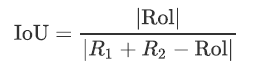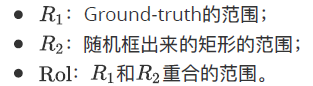## python实现IoU

### IoU公式### python实现IoU源码：

``````import numpy as np

# get IoU overlap ratio
def iou(a, b):
# get area of a
area_a = (a - a) * (a - a)
# get area of b
area_b = (b - b) * (b - b)

# get left top x of IoU
iou_x1 = np.maximum(a, b)
# get left top y of IoU
iou_y1 = np.maximum(a, b)
# get right bottom of IoU
iou_x2 = np.minimum(a, b)
# get right bottom of IoU
iou_y2 = np.minimum(a, b)

# get width of IoU
iou_w = iou_x2 - iou_x1
# get height of IoU
iou_h = iou_y2 - iou_y1

# get area of IoU
area_iou = iou_w * iou_h
# get overlap ratio between IoU and all area
iou = area_iou / (area_a + area_b - area_iou)

return iou

# [x1, y1, x2, y2]
a = np.array((50, 50, 150, 150), dtype=np.float32)

b = np.array((60, 60, 170, 160), dtype=np.float32)

print(iou(a, b))
``````